Question 1

Read the following quotation, and then research the term microcontroller to see what relevance it has to the quote:

I went to my first computer conference at the New York Hilton about 20 years ago. When somebody there predicted the market for microprocessors would eventually be in the millions, someone else said, “Where are they all going to go? It’s not like you need a computer in every doorknob!” Years later, I went back to the same hotel. I noticed the room keys had been replaced by electronic cards you slide into slots in the doors. There was a computer in every doorknob. - Danny Hillis

Question 2

A microcontroller unit, or MCU, is a specialized type of digital computer used to provide automatic sequencing or control of a system. Microcontrollers differ from ordinary digital computers in being very small (typically a single integrated circuit chip), with several dedicated pins for input and/or output of digital signals, and limited memory. Instructions programmed into the microcontroller’s memory tell it how to react to input conditions, and what types of signals to send to the outputs.

The simplest type of signal “understood” by a microcontroller is a discrete voltage level: either “high” (approximately +V) or “low” (approximately ground potential) measured at a specified pin on the chip. Transistors internal to the microcontroller produce these “high” and “low” signals at the output pins, their actions being modeled by SPDT switches for simplicity’s sake: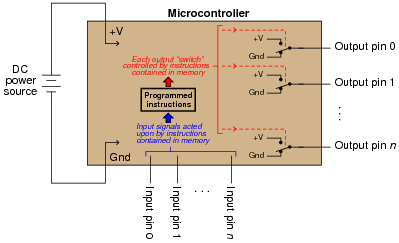Microcontrollers may be programmed to emulate the functions of digital logic gates (AND, OR, NAND, NOR, etc.) in addition to a wide variety of combinational and multivibrator functions. The only real limits to what a microcontroller can do are memory (how large of a program may be stored) and input/output pins on the MCU chip.

However, microcontrollers are themselves made up of many thousands (or millions!) of logic gate circuits. Why would it make sense to use a microcontroller to perform a logic function that a small fraction of its constituent gates could accomplish directly? In other words, why would anyone bother to program a microcontroller to perform a digital function when they could build the logic network they needed out of fewer gate circuits?

Question 3

A student decides to build a light-flasher circuit using a microcontroller instead of a 555 timer or some other hard-wired astable circuit. Unfortunately, there is a problem somewhere. When first powered up, the LED lights on for 1 second, then turns off and never turns back on. The only way the LED ever comes back on is if the MCU is reset or its power is cycled off and on: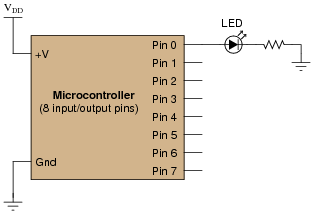Pseudocode listing Declare Pin0 as an output

BEGIN

Set Pin0 HIGH

Pause for 1 second

Set Pin0 LOW

END

A fellow student, when asked for help, modifies the program listing and re-sends it from the personal computer where it is being edited to the microcontroller, through a programming cable. The program listing now reads as such:

Pseudocode listing Declare Pin0 as an output

LOOP

Set Pin0 HIGH

Pause for 1 second

Set Pin0 LOW

ENDLOOP

When the MCU is reset with the new program, the LED starts blinking on and off . . . sort of. The LED is “on” most of the time, but once every second it turns off and then immediately comes back on. In fact, the “off” period is so brief it is barely noticeable.

What the student wanted was a 50% duty cycle: “on” for 1 second, then “off” for 1 second, repeating that cycle indefinitely. First, explain the significance of the classmate’s program modification, and then modify the program listing again so that the LED does what the student wants it to.

Question 4

A student decides to build a light-flasher circuit using a microcontroller. The LED is supposed to blink on and off only when the pushbutton switch is depressed. It is supposed to turn off when the switch is released: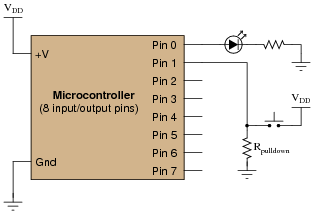Pseudocode listing Declare Pin0 as an output

Declare Pin1 as an input

WHILE Pin1 is HIGH

Set Pin0 HIGH

Pause for 0.5 seconds

Set Pin0 LOW

Pause for 0.5 seconds

ENDWHILE

The LED blinks on and off just fine as long as the pushbutton switch is held when the MCU is powered up or reset. As soon as the switch is released, the LED turns off and never comes back on. If the switch was never pressed during start-up, the LED never comes on! Explain what is happening, and modify the program as necessary to fix this problem.

Question 5

Examine the following schematic diagram and program listing (written in “pseudocode” rather than a formal programming language) to determine what type of basic logic function is being implemented in this microcontroller unit: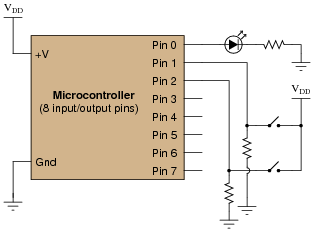Pseudocode listing Declare Pin0 as an output

Declare Pin1 and Pin2 as inputs

LOOP

IF Pin1 is HIGH, set Pin0 HIGH

ELSEIF Pin2 is HIGH, set Pin0 HIGH

ELSE set Pin0 LOW

ENDIF

ENDLOOP

Question 6

Examine the following schematic diagram and program listing (written in “pseudocode” rather than a formal programming language) to determine what type of basic logic function is being implemented in this microcontroller unit:Pseudocode listing Declare Pin0 as an output

Declare Pin1 and Pin2 as inputs

LOOP

IF Pin1 is LOW, set Pin0 LOW

ELSEIF Pin2 is LOW, set Pin0 LOW

ELSE set Pin0 HIGH

ENDIF

ENDLOOP

Question 7

Examine the following schematic diagram and program listing (written in “pseudocode” rather than a formal programming language) to determine what type of basic logic function is being implemented in this microcontroller unit: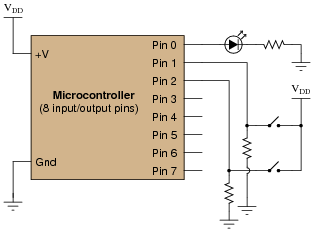Pseudocode listing Declare Pin0 as an output

Declare Pin1 and Pin2 as inputs

LOOP

IF Pin1 is LOW, set Pin0 HIGH

ELSEIF Pin2 is LOW, set Pin0 HIGH

ELSE set Pin0 LOW

ENDIF

ENDLOOP

Question 8

Examine the following schematic diagram and program listing (written in “pseudocode” rather than a formal programming language) to determine what type of basic logic function is being implemented in this microcontroller unit:Pseudocode listing Declare Pin0 as an output

Declare Pin1 and Pin2 as inputs

LOOP

IF Pin1 is HIGH, set Pin0 LOW

ELSEIF Pin2 is HIGH, set Pin0 LOW

ELSE set Pin0 HIGH

ENDIF

ENDLOOP

Question 9

Examine the following schematic diagram and program listing (written in “pseudocode” rather than a formal programming language) to determine what type of basic logic function is being implemented in this microcontroller unit: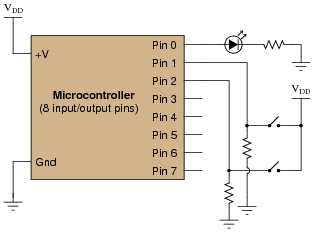Pseudocode listing Declare Pin0 as an output

Declare Pin1 and Pin2 as inputs

LOOP

IF Pin1 is same as Pin2, set Pin0 LOW

ELSE set Pin0 HIGH

ENDIF

ENDLOOP

Question 10

A microcontroller is a specialized type of digital computer used to provide automatic sequencing or control of a system. Microcontrollers differ from ordinary digital computers in being very small (typically a single integrated circuit chip), with several dedicated pins for input and/or output of digital signals, and limited memory. Instructions programmed into the microcontroller’s memory tell it how to react to input conditions, and what types of signals to send to the outputs.

The simplest type of signal “understood” by a microcontroller is a discrete voltage level: either “high” (approximately +V) or “low” (approximately ground potential) measured at a specified pin on the chip. Transistors internal to the microcontroller produce these “high” and “low” signals at the output pins, their actions being modeled by SPDT switches for simplicity’s sake: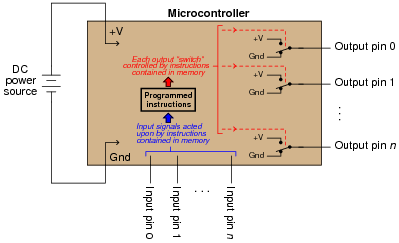It does not require much imagination to visualize how microcontrollers may be used in practical systems: turning external devices on and off according to input pin and/or time conditions. Examples include appliance control (oven timers, temperature controllers), automotive engine control (fuel injectors, ignition timing, self-diagnostic systems), and robotics (servo actuation, sensory processing, navigation logic). In fact, if you live in an industrialized nation, you probably own several dozen microcontrollers (embedded in various devices) and don’t even realize it!

One of the practical limitations of microcontrollers, though, is their low output drive current limit: typically less than 50 mA. The miniaturization of the microcontroller’s internal circuitry prohibits the inclusion of output transistors having any significant power rating, and so we must connect transistors to the output pins in order to drive any significant load(s).

Suppose we wished to have a microcontroller drive a DC-actuated solenoid valve requiring 2 amps of current at 24 volts. A simple solution would be to use an NPN transistor as an “interposing” device between the microcontroller and the solenoid valve like this: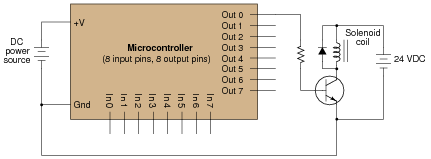Unfortunately, a single BJT does not provide enough current gain to actuate the solenoid. With 20 mA of output current from the microcontroller pin and a β of only 25 (typical for a power transistor), this only provides about 500 mA to the solenoid coil.

A solution to this problem involves two bipolar transistors in a Darlington pair arrangement: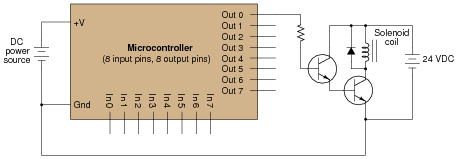However, there is another solution yet - replace the single BJT with a single MOSFET, which requires no drive current at all. Show how this may be done: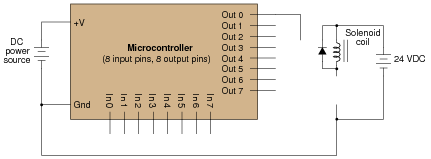Question 11

A microcontroller is used to provide automatic power factor correction for an AC load:Examine this schematic diagram, then answer the following questions:

How can the microcontroller sense the power factor of the AC load?
How many discrete steps of power factor correction can the microcontroller engage through its four output pins?
What would the MCU’s output be to correct for a load drawing 15 amps with a lagging power factor of 0.77? Assume a line frequency of 60 Hz, and a correction algorithm that adjusts for the best lagging power factor (i.e. it will never over-correct and produce a leading power factor).
What is the corrected (total) power factor with those capacitors engaged?

Question 12

This microcontroller is programmed to vary the perceived brightness of an LED by means of pulse-width modulation (PWM) control of pin 0’s output: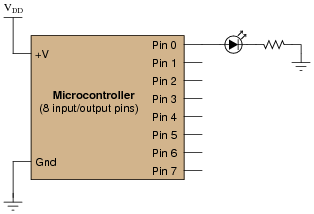Pseudocode listing Declare Pin0 as an output

Declare X as an integer variable

LOOP

Set Pin0 LOW

Pause for 100 - X microseconds

Set Pin0 HIGH

Pause for X microseconds

ENDLOOP

Determine what the value of X must be to set the LED’s brightness at 80%, and also what the frequency of the PWM signal is.

Question 13

Many microcontrollers come equipped with a built-in PWM function, so that you do not have to code a custom PWM algorithm yourself. This fact points to the popularity of pulse-width modulation as a control scheme. Explain why PWM is so popular, and give a few practical examples of how it may be used.

Question 14

Pulse-width modulation (PWM) is not only useful for generating an analog output with a microcontroller, but it is also useful for receiving an analog input through a pin that only handles on-off (high-low) digital voltage levels. The following circuit takes an analog voltage signal in to a comparator, generates PWM, then sends that PWM signal to the input of a microcontroller: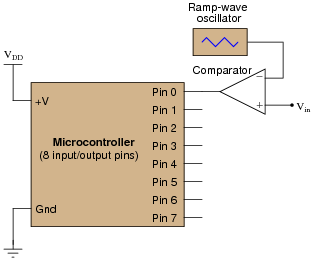Pseudocode listing Declare Pin0 as an input

Declare Last_Pin0 as a boolean variable

Declare Time_High as an integer variable

Declare Time_Low as an integer variable

Declare Duty_Cycle as a floating-point variable

Set Time_High and Time_Low both to zero

LOOP

Set Last_Pin0 equal to Pin0

If Pin0 is HIGH, increment Time_High by one

If Pin0 is LOW, increment Time_Low by one

If Last_Pin0 is not equal to Pin0, go to SUBROUTINE

ENDLOOP

SUBROUTINE

Set Duty_Cycle equal to (Time_High / (Time_High + Time_Low))

Set Time_High and Time_Low both to zero

ENDSUBROUTINE

Explain how this program works. Hint: the Last_Pin0 boolean variable is used to detect when the state of Pin0 has changed from 0 to 1 or from 1 to 0.

Question 15

Digital computers communicate with external devices through ports: sets of terminals usually arranged in groups of 4, 8, 16, or more (4 bits = 1 nybble, 8 bits = 1 byte, 16 bits = 2 bytes). These terminals may be set to high or low logic states by writing a program for the computer that sends a numerical value to the port. For example, here is an illustration of a microcontroller being instructed to send the hexadecimal number F3 to port A and 2C to port B: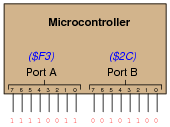Suppose we wished to use the upper four bits of port A (pins 7, 6, 5, and 4) to drive the coils of a stepper motor in this eight-step sequence:

Step 1:
0001
Step 2:
0011
Step 3:
0010
Step 4:
0110
Step 5:
0100
Step 6:
1100
Step 7:
1000
Step 8:
1001

As each pin goes high, it drives a power MOSFET on, which sends current through that respective coil of the stepper motor. By following a “shift” sequence as shown, the motor will rotate a small amount for each cycle.

Write the necessary sequence of numbers to be sent to port A to generate this specific order of bit shifts, in hexadecimal. Leave the lower four bit of port A all in the low logic state.

Question 16

Examine the following schematic diagram and program listing (written in “pseudocode” rather than a formal programming language) to determine what type of basic logic function is being implemented in this microcontroller unit:Pseudocode listing Declare Pin0 as an output

Declare Pin1 and Pin2 as inputs

LOOP

IF Pin1 is same as Pin2, set Pin0 HIGH

ELSE set Pin0 LOW

ENDIF

ENDLOOP

Question 17

Digital computers communicate with external devices through ports: sets of terminals usually arranged in groups of 4, 8, 16, or more. These terminals may be set to high or low logic states by writing a program for the computer that sends a numerical value to the port. For example, here is an illustration of a microcontroller being instructed to send the hexadecimal number 2B to port A and A9 to port B: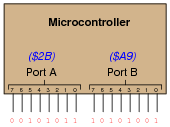Suppose we wished to use the first seven bits of each port (pins 0 through 6) to drive two 7-segment, common-cathode displays, rather than use BCD-to-7-segment decoder ICs: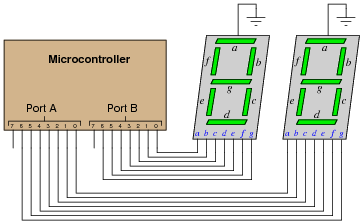Write the necessary hexadecimal values to be output at ports A and B to generate the display “42” at the two 7-segment display units.

Question 18

One method of driving pixels in a grid-based display is to organize the pixels into rows and columns, then select individual pixels for illumination by the intersection of a specific row line and a specific column line. In this example, we are controlling an 8 × 8 grid of LEDs with two 8-bit (1-byte) ports of a microcontroller: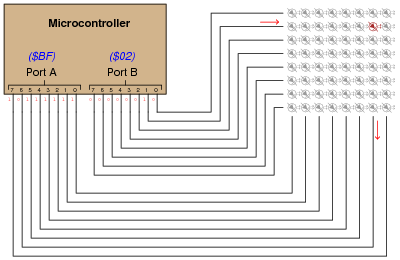Note that a high state is required on one of port B’s pins to activate a row, and a low state is required on one of port A’s pins to activate a column, because the LED anodes connect to port A and the LED cathodes connect to port B.

Determine the hexadecimal codes we would need to output at ports A and B to energize the LED in the far lower-left corner of the 8 × 8 grid.

Port A =

Port B =

Question 19

Examine the following schematic diagram and program listing (written in “pseudocode” rather than a formal programming language) to determine what type of basic logic function is being implemented in this microcontroller unit: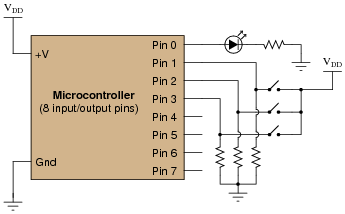Pseudocode listing Declare Pin0 as an output

Declare Pin1, Pin2, and Pin3 as inputs

LOOP

IF Pin1 is HIGH, set Pin0 HIGH

ELSEIF Pin2 is HIGH, set Pin0 HIGH

ELSEIF Pin3 is HIGH, set Pin0 HIGH

ELSE set Pin0 LOW

ENDIF

ENDLOOP

Question 20

A student builds a microcontroller circuit to turn on an LED once for every five actuations of the input switch. The circuit is simple, with the microcontroller using a conditional loop to increment a variable each time the switch is pressed:Pseudocode listing Declare Pin0 as an output

Declare Pin1 as an input

Declare X as an integer variable

LOOP

WHILE Pin1 is HIGH

Add 1 to X (X=X+1)

ENDWHILE

IF X is equal to 5, set Pin0 HIGH and set X to 0

ELSE set Pin0 LOW

ENDIF

ENDLOOP

Unfortunately, the program does not execute as planned. Instead of the LED coming on once every five switch actuations, it seems to come on randomly when the switch is released. Sometimes the LED turns on after the first switch actuation, while other times it takes more than five pushes of the switch to get it to turn on.

After some careful analysis, it occurs to the student that the problem lies in the WHILE loop. As the microcontroller is much faster than the human hand, that loop executes many times while the switch is being pressed rather than only once, meaning that the variable X counts from 0 to 5 many times around for each switch actuation. It is only by chance, then, that X will be equal to five after the WHILE loop exits.

What the student needs is for the switch to increment by 1 only for an off-to-on switch transition: at the positive edge of the input pulse. The problem is how to do this using programming.

Another student, when faced with the same problem, chose to solve it this way and it worked just fine:

Pseudocode listing Declare Pin0 as an output

Declare Pin1 as an input

Declare Switch as a Boolean (0 or 1) variable

Declare Last_Switch as a Boolean (0 or 1) variable

Declare X as an integer variable

LOOP

Set Last_Switch equal to Switch

Set Switch equal to Pin1

IF Switch = 1 and Last_Switch = 0 THEN add 1 to X (X=X+1)

ELSE do nothing to X

ENDIF

IF X is equal to 5, set Pin0 HIGH and set X to 0

ELSE set Pin0 LOW

ENDIF

ENDLOOP

Explain how this program successfully increments X only on each off-to-on transition of the pushbutton switch while the other program increments X rapidly during the entire duration the pushbutton switch is pressed.

Related Tools:

Published under the terms and conditions of the Creative Commons Attribution License